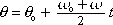theta =  theta-sub-zero    plus    [ (omega-sub-zero plus omega) over 2 ] times t

This is one of the constant angular acceleration equations. It's the one with no "alpha" in it.

theta [radians] is the angular position of the object at time t.
theta-sub-zero [radians] is the angular position of the object at time 0.
omega-sub-zero [radians-per-second] is the angular velocity of the object at time 0.
omega [radians-per-second] is the angular velocity of the object at time t.
t [seconds] is the time.

Tip: Omega is how fast the object is spinning. You want it in radians per second but it is often given in revolutions per second or in rpm which means "revolutions per minute". Convert it to radians per second.

Pitfall Avoidance Note: Omega does not have units of m/s.